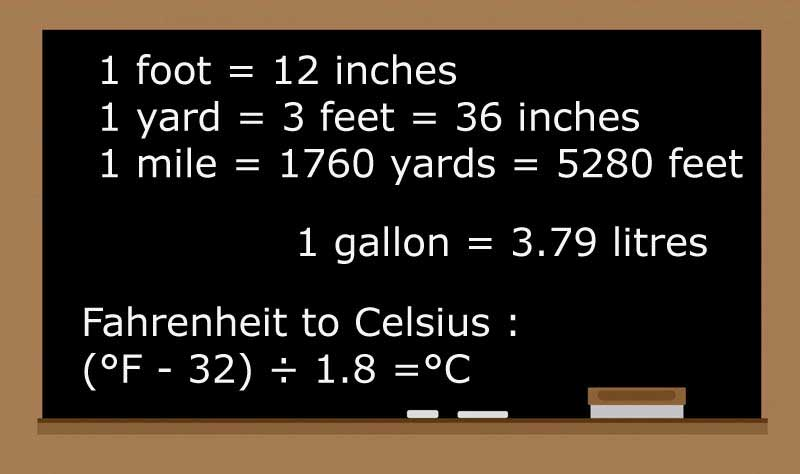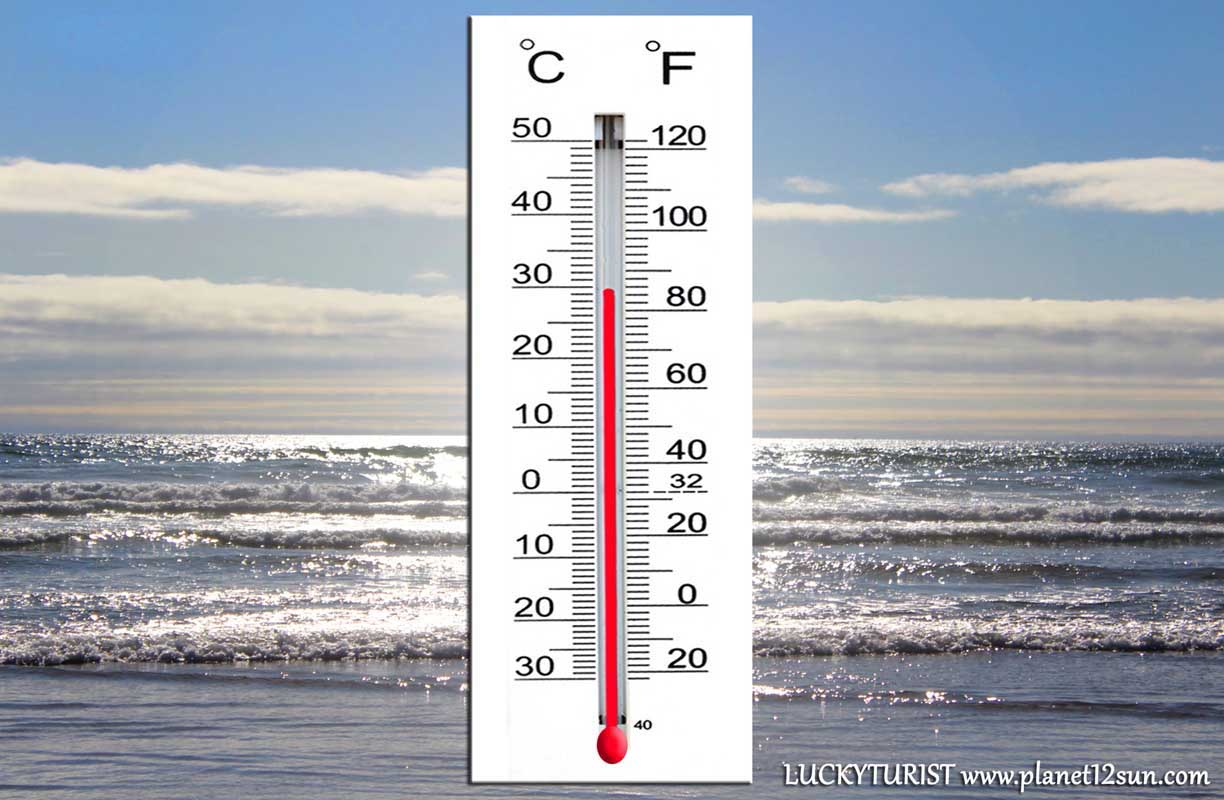## Temperature

Temperature is how hot or cold a thing is. It is measured by a thermometer. Thermometers use a temperature scale, which is most often used in degrees Celsius (°C), sometimes called centigrade. In the USA, degrees Fahrenheit (°F) are more often used while scientists mostly use kelvins (K) to measure temperature because it never goes below zero.### Celsius

The Celsius scale (°C) is used for common temperature measurements in most of the world. It is an empirical scale. It developed by a historical progress, which led to its zero point 0°C being defined by the freezing point of water, with additional degrees defined so that 100°C was the boiling point of water, both at sea-level atmospheric pressure.

Useful temperatures:

On the Celsius scale, water freezes at 0° and boils at 100°.
Room temperature is about 20 °C.
A hot sunny day might have a temperature of 30°C.
Absolute zero (the coldest possible temperature) is -273.15 °C.
Winters in Antarctica can be between -80 and -90 °C.
A human's body temperature is usually 37 °C.
You can bake cookies in your oven at a temperature of 180°C.

### Fahrenheit

The United States commonly uses the Fahrenheit scale, on which water freezes at 32 °F and boils at 212 °F at sea-level atmospheric pressure. The degree Fahrenheit is often considered to be "old fashioned" throughout the majority of the world as it is an older and outdated way of measuring temperature.

Useful temperatures:

Water freezes at 32 °F.
The temperature inside the human body is usually 98 °F.
Water boils at 212 °F.
The coldest possible temperature is absolute zero. Absolute zero is -459 °F.
A hot sunny day might have a temperature of 85°F.
You can bake cookies in your oven at a temperature of 350°F.

### Conversion of Temperature

from Celsius to Fahrenheit: first multiply by 1.8, then add 32
from Fahrenheit to Celsius: first subtract 32, then multiply by 1.8

Celsius to Fahrenheit : (°C × 1.8) + 32 =°F
Fahrenheit to Celsius : (°F - 32) ÷ 1.8 =°C

from Celsius to Celsius
Fahrenheit [°F] = [°C] × 9/5  + 32 [°C] = ([°F] - 32) × 5/9
Kelvin [K] = [°C] + 273.15 [°C] = [K] - 273.15
Newton [°N] = [°C] × 33/100 [°C] = [°N] × 100/33